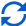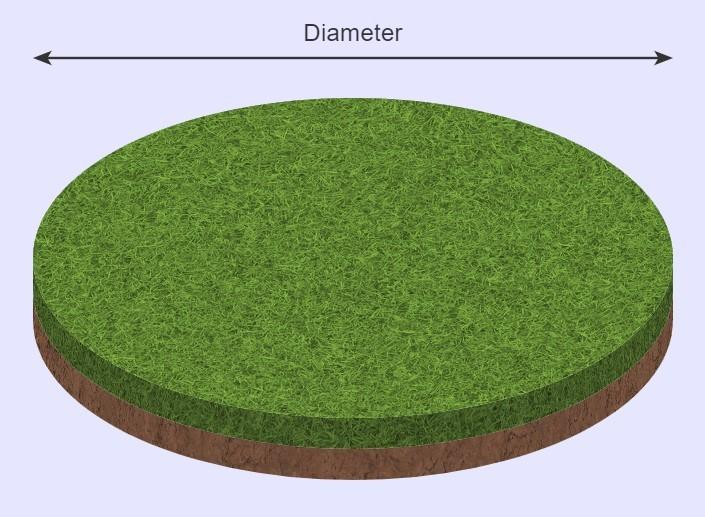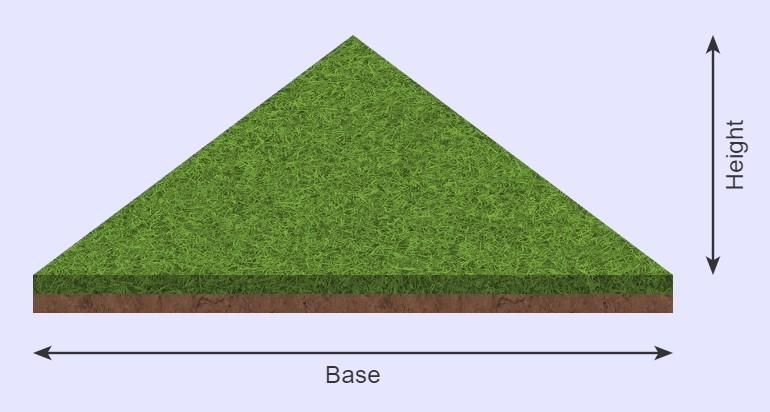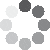Enter parameters Square
Enter parameters
Length:
ft
• inches (in)
• feet (ft)
• yards (yd)
• centimeters (cm)
• meters (m)
Width:
ft
• inches (in)
• feet (ft)
• yards (yd)
• centimeters (cm)
• meters (m)
Price:
pallet
• Per Pallet - 500 ft² (pallet)
• Per Roll - 10 ft² (roll)
• Per square feet (ft²)
• Per square inches (in²)
• Per square yards (yd²)
• Per acres (ac)
• Per square meters ()Result
Area:
0
ft²
• square feet (ft²)
• square inches (in²)
• square yards (yd²)
• square miles (mi²)
• acres (ac)
• square centimeters (cm²)
• square meters ()
Сost:
$Pallets: 0 Enter parameters Diameter: ft • inches (in) • feet (ft) • yards (yd) • centimeters (cm) • meters (m) Price: pallet • Per Pallet - 500 ft² (pallet) • Per Roll - 10 ft² (roll) • Per square feet (ft²) • Per square inches (in²) • Per square yards (yd²) • Per acres (ac) • Per square meters ()Result Area: 0 ft² • square feet (ft²) • square inches (in²) • square yards (yd²) • square miles (mi²) • acres (ac) • square centimeters (cm²) • square meters () Сost:$
Pallets:
0
Enter parameters
Base:
ft
• inches (in)
• feet (ft)
• yards (yd)
• centimeters (cm)
• meters (m)
Height:
ft
• inches (in)
• feet (ft)
• yards (yd)
• centimeters (cm)
• meters (m)
Price:
pallet
• Per Pallet - 500 ft² (pallet)
• Per Roll - 10 ft² (roll)
• Per square feet (ft²)
• Per square inches (in²)
• Per square yards (yd²)
• Per acres (ac)
• Per square meters ()Result
Area:
0
ft²
• square feet (ft²)
• square inches (in²)
• square yards (yd²)
• square miles (mi²)
• acres (ac)
• square centimeters (cm²)
• square meters ()
Сost:
$Pallets: 0(2 votes, average: 4.50 out of 5)Loading... Similar Calculators: Real-time graphics. Make the calculations and see the changes. Real-time graphics. Make the calculations and see the changes. Real-time graphics. Make the calculations and see the changes. Embed Similar Calculators:The key to any successful home improvement or landscaping project is preparation. Having an accurate idea of how much material you will need is vital. Guessing how many pallets of sod your garden will require, or how many square feet of grass you will need to ensure equal coverage is not easy. Don’t worry – you can use our free online sod calculator to work this out for you. 😉 In this article we will show you just how simple it is to use and how it can help you efficiently calculate how many rolls of sod you will need to purchase. In addition, we will explain the Mathematics the calculator uses. Contents: ## Sod calculator formula The calculator estimates the total area of the sod required based on the measurements you provide. These can be in metric units (centimeters or meters) or imperial units (yards, feet or inches). The total area of the sod required is calculated by the following formulae: ### Rectangular Garden BedThe total sod area of a rectangular bed is given by: $$Sod\,Area = Length × Width$$ ### Circular Garden BedFor a circular garden bed, the total sod area is calculated using the formula: $$Sod\,Area = \pi × Radius^2$$ where $$Radius = {Diameter \over 2}$$ ### Triangular Garden BedFor a triangular garden bed, the total area is calculated using the formula: $$Sod\,Area = {Height × Base \over 2}$$ NB: Sod is often sold in pieces of grass and soil, often referred to as pallets or rolls. The standard dimensions of a pallet is 500 square feet and a roll is 10 square feet. Our calculator can work out how many pallets and rolls should be purchased using the formulae: $$Number\,of\,Pallets = {Sod\,Area \over 500\,ft^2}$$ $$Number\,of\,Rolls = {Sod\,Area \over 10\,ft^2}$$ Please note that the number of pallets or rolls calculated should always be rounded up. Otherwise you may end up not purchasing sufficient sod. ### Calculating Price If you know the unit price of the sod, then the calculator can work out the total cost of the landscaping project: $$Cost = Price\,per\,Unit\,Area × Sod\,Area$$ If you don’t know the unit price of the sod, it’s easy enough to calculate for yourself: $$Price\,Per\,Unit\,Area = {Cost\,of\,Sod \over Total\,Sod\,Area}$$ Something unclear? 😕 See below for three fully worked examples where we estimate how much sod different landscaping projects will need. Otherwise, simply enter your values and measurements into the sod calculator to get your next landscaping project off to an efficient start! ## Example calculations 📌 Rectangular Garden Lawn with known price per pallet. • Let’s say that I have a garden lawn measuring 80 feet long and 50 feet wide. • I want to lay down sod which costs$250 per pallet (which is 500 square footage in dimensions).
• I want to determine how much sod is needed, how many pallets in total I should buy and an estimate for how much this landscaping project will cost.

I enter the above values into the sod area calculator to work out the Sod Area:

$$Sod\,Area = Length × Width = 80\,ft × 50\,ft = 4000\,ft^2$$

the total number of pallets I should buy:

$$Number\,of\,Pallets = {Sod\,Area \over 500\,ft^2} = {4000\,ft^2 \over 500\,ft^2} = 8\,pallets$$

and the total estimated cost of the project:

$$Cost = Price\,per\,Unit\,Area × Sod\,Area = 250\,/pallet × 8\,pallets= 2000$$

📌 Circular Garden Lawn with known price per roll.

• Let’s say that I want to lay down new sod on a circular garden lawn which measures 40 feet in diameter.
• I want to estimate how many rolls I should buy, and how much this project will cost.

Entering these measurements into the online sod calculator gives:

$$Radius = {Diameter \over 2} = {40\,ft \over 2} = 20\,ft$$

$$Sod\,Area = \pi × Radius^2 = \pi × 20\,ft^2 = 1256.6\,ft^2$$

Let’s imagine that the sod costs $15 per roll and a roll measures 10 square feet. Therefore, we can calculate the total number of pallets and rolls: $$Number\,of\,Pallets = {Sod\,Area \over 500\,ft^2} = {1256.6\,ft^2 \over 500\,ft^2} = 2.51 \rightarrow 3\,pallets$$ $$Number\,of\,Rolls = {Sod\,Area \over 10\,ft^2} = {1256.6\,ft^2 \over 10\,ft^2} = 125.6 \rightarrow 126\,rolls$$ Note how we round these values up! and the total cost: $$Cost = Price\,per\,Unit\,Area × Sod\,Area=15\,/roll × 126\,rolls = 1890$$ 📌 Triangular Garden Lawn with unknown price per unit area. • For our final example lets imagine we have a triangular garden lawn that is 50 feet in length and 80 feet in height. • I don’t know the price per square foot of the sod, but I can buy 60 square feet of sod for$24.

I enter the above measurements into the sod area calculator to work out the total sod area:

$$Sod\,Area = {Height × Base \over 2} = {50\,ft × 80\,ft \over 2} = 2000\,ft^2$$

To calculate the total cost, I would first need to work out the price per square foot:

$$Price\,Per\,Unit\,Area = {Cost\,of\,Sod \over Total\,Sod\,Area} = {24 \over 60\,ft^2} = 0.4\,/ft^2$$

Then, the sod calculator can work out an estimate for the total cost of the sod:

$$Cost = Price\,per\,Unit\,Area × Sod\,Area = 0.4\,/ft^2 × 2000\,ft^2 = 800$$

and how the total number of pallets I will need:

$$Number\,of\,Pallets = {Sod\,Area \over 500\,ft^2} = {2000\,ft^2 \over 500\,ft^2} = 4\,pallets$$

At this stage, you are probably wondering:

## What if I want to work in units other than feet?

Well, the answer couldn’t be simpler! Whether you want to work in metric units (centimeters and meters) or imperial units (feet, yards or inches), our calculator can help!

All you need to do is select the desired units in the drop down options and our calculator will do the following conversions for you:

$$1\,foot = 12\,inches = 0.33\,yards = 30.48\,centimeters = 0.3048\,meters$$

$$1\,ft^2 = 144\,in^2 = 0.111\,yd^2 = 929\,cm^2= 0.0929\,m^2$$

In addition, the price per unit volume can also be given in pallets, rolls and acres. The calculator does the following conversions for you:

$$1\,acre = 43,560\,ft^2$$

$$1\,pallet = 500\,ft^2$$

$$1\,roll = 10\,ft^2$$

It couldn’t be simpler! 🙂

Embed this calculator on your site!Add live graphics
Copied to clipboard! Preview